Next:3.10 To Probe FurtherUp:3.9 Analysis of Skip ListsPrevious:3.9 Analysis of Skip Lists

## 3.9.1 Analysis of Expected Search Cost

We analyze the search path backwards, traveling up and to the left. We pretend as if the level of a node is being determined only when it is observed while backtracking the search path.
1.
From level 1, we rise to level L(n)
2.
We move leftward
3.
We climb from L(n) to maxLevel.
At a particular point in the climb, we are at the ith forward pointer of a node x and we have no knowledge about the levels of nodes to the left of x or about the level of x (other than that the level of x must be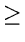i ). See situation (a) in figure.
• Assume x'' is not the header.
• If level of x is i, then we are in situation (b). If level of x is > i, we are in situation (c).
• Prob{ we are in situation cA} = p
• Each time we are in situation c, we climb up one level.
Let
 C(k) = expected length of a search path that .climbs up k levels in an infinite list.
Then:
 C(0) = 0 C(k) = p  {cost in situation c } (1 - p)  { cost in situation b }
We get

 C(k) = p  {1 + C(k - 1)} + (1 - p){1 + C(k)} C(k) =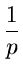+ C(k - 1) C(k) =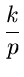Our assumption that the list is infinite is pessimistic.

When we bump into the header in our backward climb, we simply climb it up without performing any leftward (horizontal) movements.

This gives us an upperbound of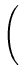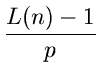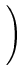On the expected length of a path that climbs up from level 1 to level L(n) in a list of n elements. (because L(n) - 1 level are being climbed).

The rest of the journey includes

1.
leftward movements, the number of which is bounded by the number of elements of level L(n)or higher. his has an expected value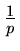2.
We also move upwards from level L(n) to the maximum level in the list.

 p  {   maximum level in the list > k} = 1 - (1 - pk)nnpk

 p  {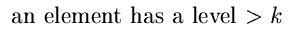} = pk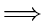p  {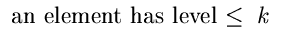} = 1 - pkp  {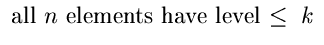} = (1 - pk)np  {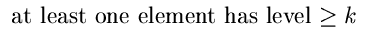} = 1 - (1 - pk)n Expected maximum levelnpkExpected maximum levelL(n) +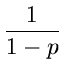Putting our results together, we get:

Total expected cost to climb out of a list on n elements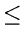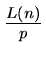which is O(log n).

We can also compute the probability if the actual cost of a search exceeds expected cost by more than a specified ratio.

Examples

1.
p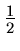;    n = 256

p  {search will take longer than 3 times the expected cost  } =2.
p;    n = 4096

p  {search will take longer than 2 times the expected cost  } =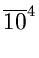3.
p;    n = 1024

p  {search will take longer than 3 times  } =How do we choose p ?
• Decreasing p increases variability of running times.
• p; generation of random level can be done from a stream of random bits.
• Decreasing p decreases # of pointers per node.
• Overheads are related to L(n) rather than.
• p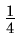is probably the best choice, unless variability of running times is an issue.Next:3.10 To Probe FurtherUp:3.9 Analysis of Skip ListsPrevious:3.9 Analysis of Skip Lists
eEL,CSA_Dept,IISc,Bangalore